Anzeige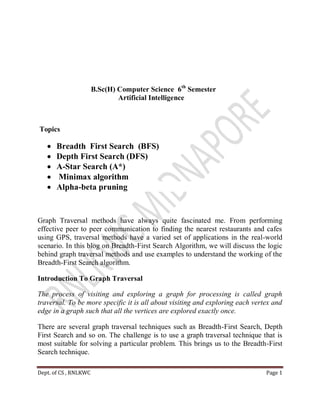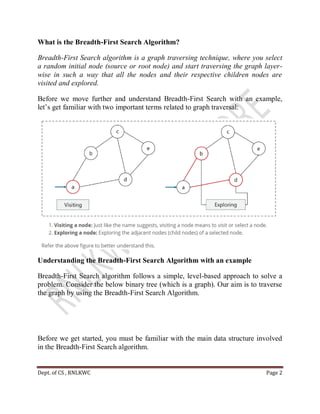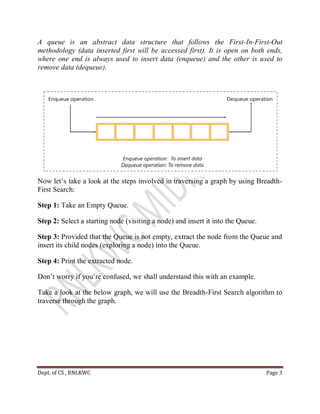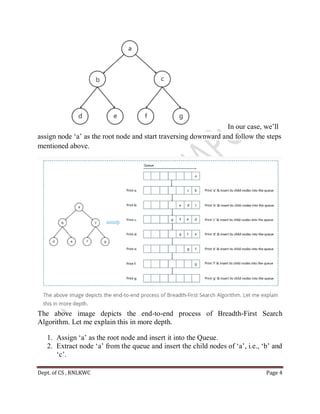Anzeige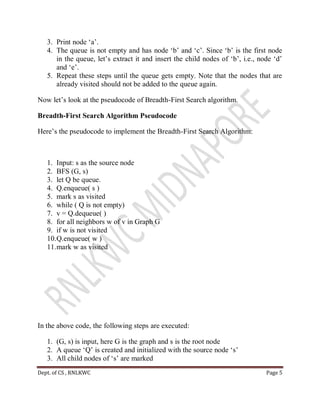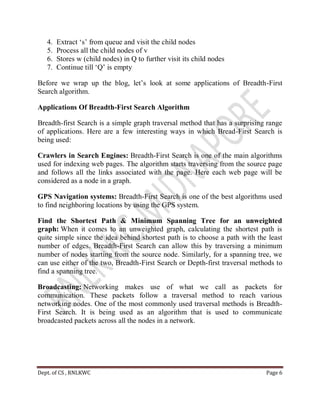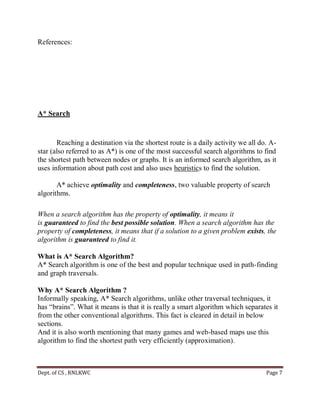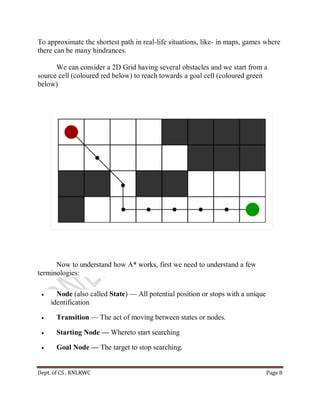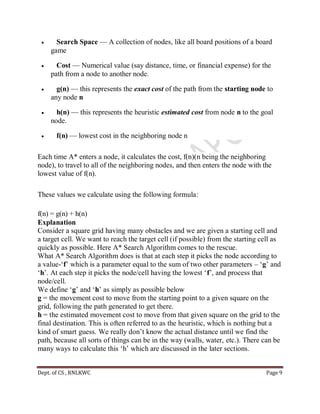Anzeige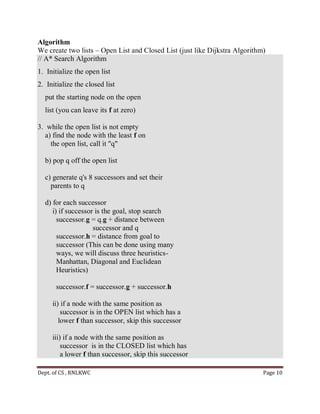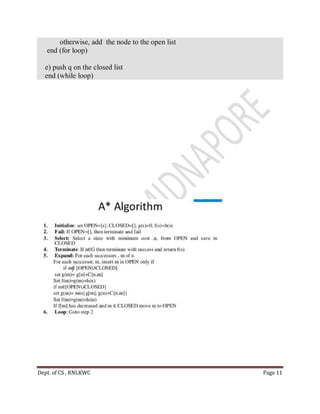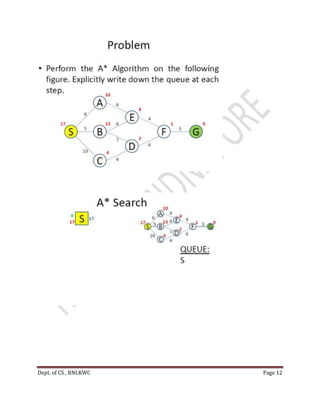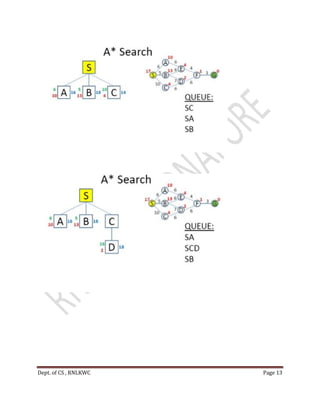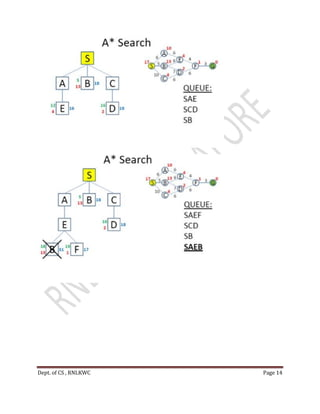Anzeige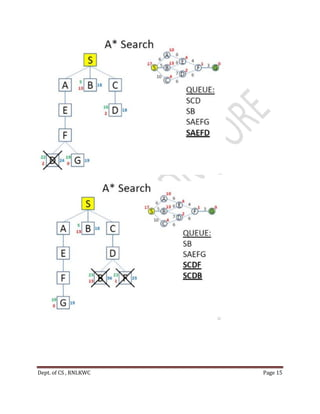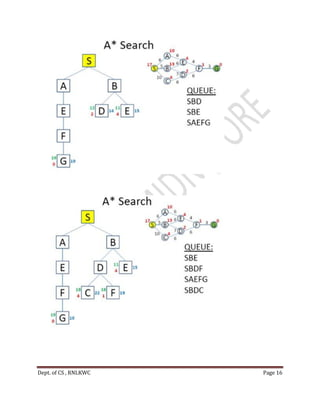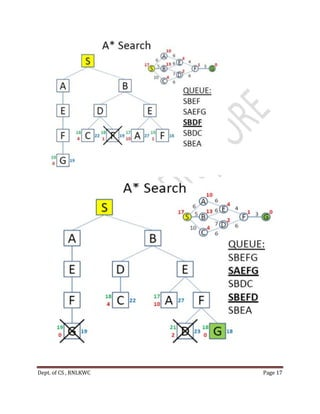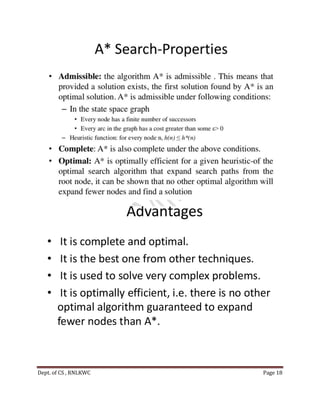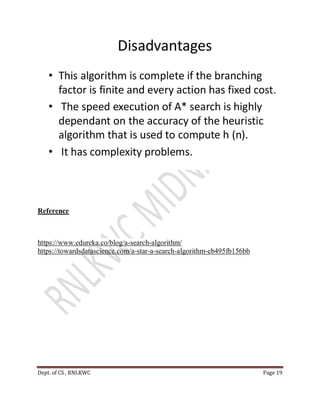Anzeige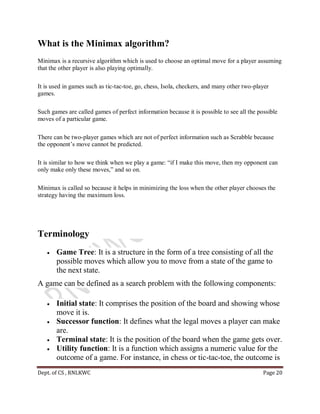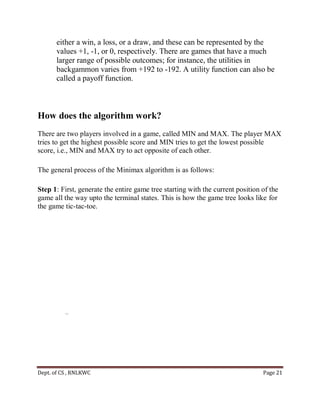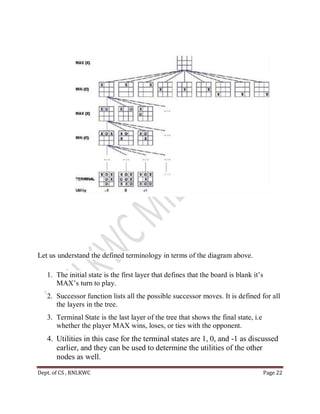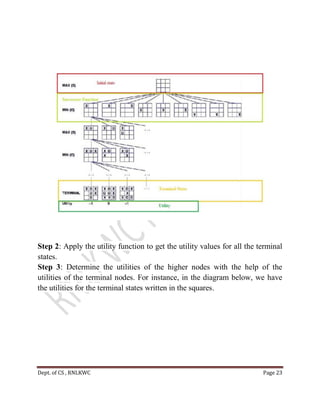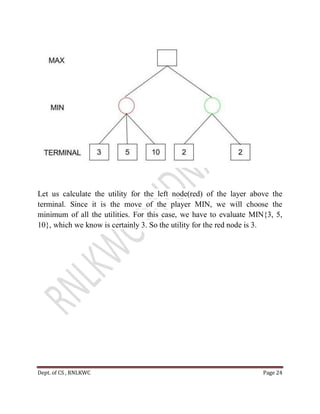Anzeige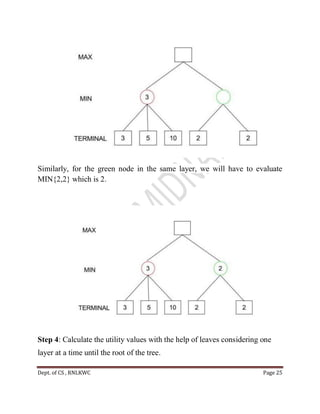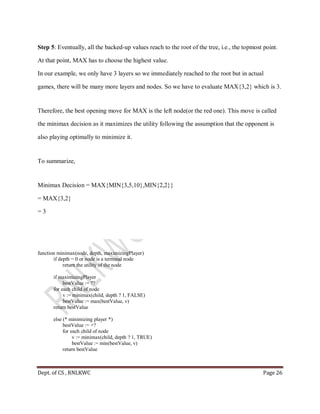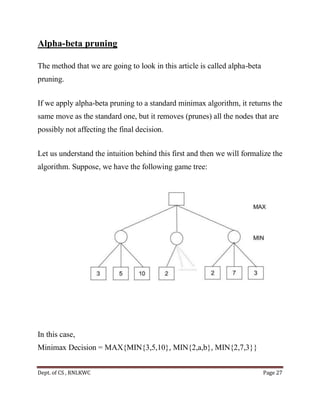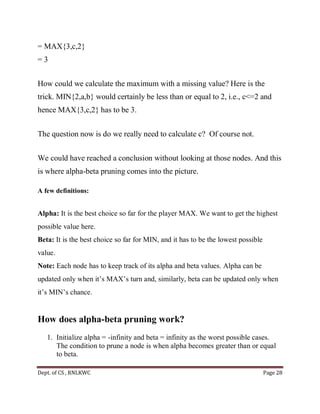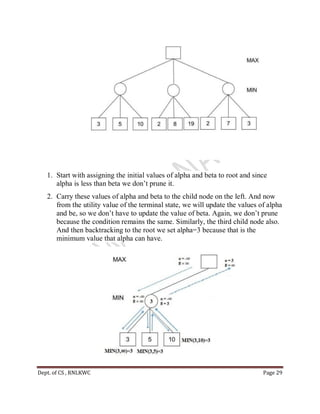Anzeige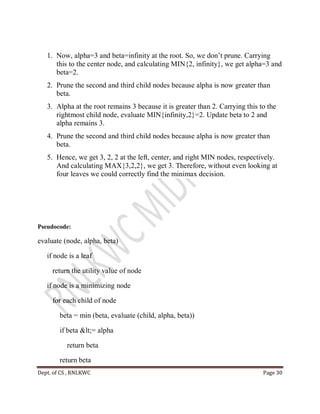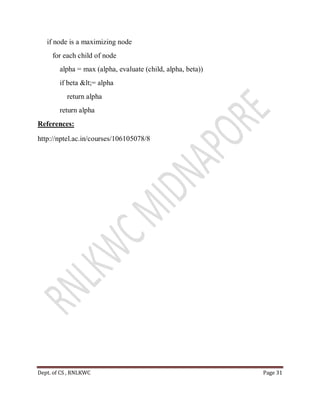Nächste SlideShare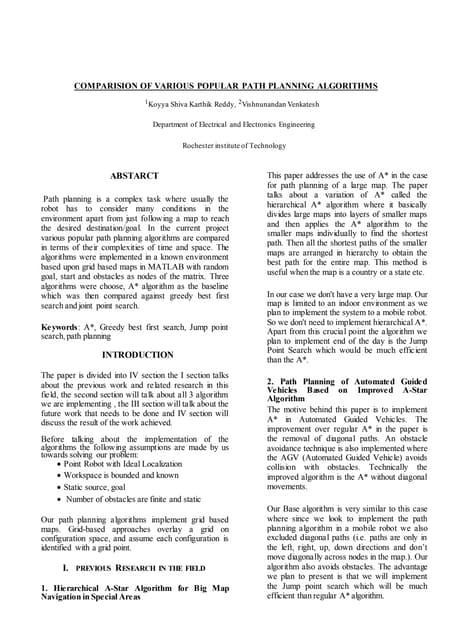artifical intelligence final paper
1 von 31
Anzeige

### Ai1.pdf

1. Dept. of CS , RNLKWC Page 1 B.Sc(H) Computer Science 6th Semester Artificial Intelligence Topics  Breadth First Search (BFS)  Depth First Search (DFS)  A-Star Search (A*)  Minimax algorithm  Alpha-beta pruning Graph Traversal methods have always quite fascinated me. From performing effective peer to peer communication to finding the nearest restaurants and cafes using GPS, traversal methods have a varied set of applications in the real-world scenario. In this blog on Breadth-First Search Algorithm, we will discuss the logic behind graph traversal methods and use examples to understand the working of the Breadth-First Search algorithm. Introduction To Graph Traversal The process of visiting and exploring a graph for processing is called graph traversal. To be more specific it is all about visiting and exploring each vertex and edge in a graph such that all the vertices are explored exactly once. There are several graph traversal techniques such as Breadth-First Search, Depth First Search and so on. The challenge is to use a graph traversal technique that is most suitable for solving a particular problem. This brings us to the Breadth-First Search technique.
2. Dept. of CS , RNLKWC Page 2 What is the Breadth-First Search Algorithm? Breadth-First Search algorithm is a graph traversing technique, where you select a random initial node (source or root node) and start traversing the graph layer- wise in such a way that all the nodes and their respective children nodes are visited and explored. Before we move further and understand Breadth-First Search with an example, let‘s get familiar with two important terms related to graph traversal: Understanding the Breadth-First Search Algorithm with an example Breadth-First Search algorithm follows a simple, level-based approach to solve a problem. Consider the below binary tree (which is a graph). Our aim is to traverse the graph by using the Breadth-First Search Algorithm. Before we get started, you must be familiar with the main data structure involved in the Breadth-First Search algorithm.
3. Dept. of CS , RNLKWC Page 3 A queue is an abstract data structure that follows the First-In-First-Out methodology (data inserted first will be accessed first). It is open on both ends, where one end is always used to insert data (enqueue) and the other is used to remove data (dequeue). Now let‘s take a look at the steps involved in traversing a graph by using Breadth- First Search: Step 1: Take an Empty Queue. Step 2: Select a starting node (visiting a node) and insert it into the Queue. Step 3: Provided that the Queue is not empty, extract the node from the Queue and insert its child nodes (exploring a node) into the Queue. Step 4: Print the extracted node. Don‘t worry if you‘re confused, we shall understand this with an example. Take a look at the below graph, we will use the Breadth-First Search algorithm to traverse through the graph.
4. Dept. of CS , RNLKWC Page 4 In our case, we‘ll assign node ‗a‘ as the root node and start traversing downward and follow the steps mentioned above. The above image depicts the end-to-end process of Breadth-First Search Algorithm. Let me explain this in more depth. 1. Assign ‗a‘ as the root node and insert it into the Queue. 2. Extract node ‗a‘ from the queue and insert the child nodes of ‗a‘, i.e., ‗b‘ and ‗c‘.
5. Dept. of CS , RNLKWC Page 5 3. Print node ‗a‘. 4. The queue is not empty and has node ‗b‘ and ‗c‘. Since ‗b‘ is the first node in the queue, let‘s extract it and insert the child nodes of ‗b‘, i.e., node ‗d‘ and ‗e‘. 5. Repeat these steps until the queue gets empty. Note that the nodes that are already visited should not be added to the queue again. Now let‘s look at the pseudocode of Breadth-First Search algorithm. Breadth-First Search Algorithm Pseudocode Here‘s the pseudocode to implement the Breadth-First Search Algorithm: 1. Input: s as the source node 2. BFS (G, s) 3. let Q be queue. 4. Q.enqueue( s ) 5. mark s as visited 6. while ( Q is not empty) 7. v = Q.dequeue( ) 8. for all neighbors w of v in Graph G 9. if w is not visited 10.Q.enqueue( w ) 11.mark w as visited In the above code, the following steps are executed: 1. (G, s) is input, here G is the graph and s is the root node 2. A queue ‗Q‘ is created and initialized with the source node ‗s‘ 3. All child nodes of ‗s‘ are marked
7. Dept. of CS , RNLKWC Page 7 References: A* Search Reaching a destination via the shortest route is a daily activity we all do. A- star (also referred to as A*) is one of the most successful search algorithms to find the shortest path between nodes or graphs. It is an informed search algorithm, as it uses information about path cost and also uses heuristics to find the solution. A* achieve optimality and completeness, two valuable property of search algorithms. When a search algorithm has the property of optimality, it means it is guaranteed to find the best possible solution. When a search algorithm has the property of completeness, it means that if a solution to a given problem exists, the algorithm is guaranteed to find it. What is A* Search Algorithm? A* Search algorithm is one of the best and popular technique used in path-finding and graph traversals. Why A* Search Algorithm ? Informally speaking, A* Search algorithms, unlike other traversal techniques, it has ―brains‖. What it means is that it is really a smart algorithm which separates it from the other conventional algorithms. This fact is cleared in detail in below sections. And it is also worth mentioning that many games and web-based maps use this algorithm to find the shortest path very efficiently (approximation).
8. Dept. of CS , RNLKWC Page 8 To approximate the shortest path in real-life situations, like- in maps, games where there can be many hindrances. We can consider a 2D Grid having several obstacles and we start from a source cell (coloured red below) to reach towards a goal cell (coloured green below) Now to understand how A* works, first we need to understand a few terminologies:  Node (also called State) — All potential position or stops with a unique identification  Transition — The act of moving between states or nodes.  Starting Node — Whereto start searching  Goal Node — The target to stop searching.
9. Dept. of CS , RNLKWC Page 9  Search Space — A collection of nodes, like all board positions of a board game  Cost — Numerical value (say distance, time, or financial expense) for the path from a node to another node.  g(n) — this represents the exact cost of the path from the starting node to any node n  h(n) — this represents the heuristic estimated cost from node n to the goal node.  f(n) — lowest cost in the neighboring node n Each time A* enters a node, it calculates the cost, f(n)(n being the neighboring node), to travel to all of the neighboring nodes, and then enters the node with the lowest value of f(n). These values we calculate using the following formula: f(n) = g(n) + h(n) Explanation Consider a square grid having many obstacles and we are given a starting cell and a target cell. We want to reach the target cell (if possible) from the starting cell as quickly as possible. Here A* Search Algorithm comes to the rescue. What A* Search Algorithm does is that at each step it picks the node according to a value-‗f‘ which is a parameter equal to the sum of two other parameters – ‗g‘ and ‗h‘. At each step it picks the node/cell having the lowest ‗f‘, and process that node/cell. We define ‗g‘ and ‗h‘ as simply as possible below g = the movement cost to move from the starting point to a given square on the grid, following the path generated to get there. h = the estimated movement cost to move from that given square on the grid to the final destination. This is often referred to as the heuristic, which is nothing but a kind of smart guess. We really don‘t know the actual distance until we find the path, because all sorts of things can be in the way (walls, water, etc.). There can be many ways to calculate this ‗h‘ which are discussed in the later sections.
10. Dept. of CS , RNLKWC Page 10 Algorithm We create two lists – Open List and Closed List (just like Dijkstra Algorithm) // A* Search Algorithm 1. Initialize the open list 2. Initialize the closed list put the starting node on the open list (you can leave its f at zero) 3. while the open list is not empty a) find the node with the least f on the open list, call it "q" b) pop q off the open list c) generate q's 8 successors and set their parents to q d) for each successor i) if successor is the goal, stop search successor.g = q.g + distance between successor and q successor.h = distance from goal to successor (This can be done using many ways, we will discuss three heuristics- Manhattan, Diagonal and Euclidean Heuristics) successor.f = successor.g + successor.h ii) if a node with the same position as successor is in the OPEN list which has a lower f than successor, skip this successor iii) if a node with the same position as successor is in the CLOSED list which has a lower f than successor, skip this successor
11. Dept. of CS , RNLKWC Page 11 otherwise, add the node to the open list end (for loop) e) push q on the closed list end (while loop)
12. Dept. of CS , RNLKWC Page 12
13. Dept. of CS , RNLKWC Page 13
14. Dept. of CS , RNLKWC Page 14
15. Dept. of CS , RNLKWC Page 15
16. Dept. of CS , RNLKWC Page 16
17. Dept. of CS , RNLKWC Page 17
18. Dept. of CS , RNLKWC Page 18
19. Dept. of CS , RNLKWC Page 19 Reference https://www.edureka.co/blog/a-search-algorithm/ https://towardsdatascience.com/a-star-a-search-algorithm-eb495fb156bb
20. Dept. of CS , RNLKWC Page 20 What is the Minimax algorithm? Minimax is a recursive algorithm which is used to choose an optimal move for a player assuming that the other player is also playing optimally. It is used in games such as tic-tac-toe, go, chess, Isola, checkers, and many other two-player games. Such games are called games of perfect information because it is possible to see all the possible moves of a particular game. There can be two-player games which are not of perfect information such as Scrabble because the opponent‘s move cannot be predicted. It is similar to how we think when we play a game: ―if I make this move, then my opponent can only make only these moves,‖ and so on. Minimax is called so because it helps in minimizing the loss when the other player chooses the strategy having the maximum loss. Terminology  Game Tree: It is a structure in the form of a tree consisting of all the possible moves which allow you to move from a state of the game to the next state. A game can be defined as a search problem with the following components:  Initial state: It comprises the position of the board and showing whose move it is.  Successor function: It defines what the legal moves a player can make are.  Terminal state: It is the position of the board when the game gets over.  Utility function: It is a function which assigns a numeric value for the outcome of a game. For instance, in chess or tic-tac-toe, the outcome is
21. Dept. of CS , RNLKWC Page 21 either a win, a loss, or a draw, and these can be represented by the values +1, -1, or 0, respectively. There are games that have a much larger range of possible outcomes; for instance, the utilities in backgammon varies from +192 to -192. A utility function can also be called a payoff function. How does the algorithm work? There are two players involved in a game, called MIN and MAX. The player MAX tries to get the highest possible score and MIN tries to get the lowest possible score, i.e., MIN and MAX try to act opposite of each other. The general process of the Minimax algorithm is as follows: Step 1: First, generate the entire game tree starting with the current position of the game all the way upto the terminal states. This is how the game tree looks like for the game tic-tac-toe.
22. Dept. of CS , RNLKWC Page 22 Let us understand the defined terminology in terms of the diagram above. 1. The initial state is the first layer that defines that the board is blank it‘s MAX‘s turn to play. 2. Successor function lists all the possible successor moves. It is defined for all the layers in the tree. 3. Terminal State is the last layer of the tree that shows the final state, i.e whether the player MAX wins, loses, or ties with the opponent. 4. Utilities in this case for the terminal states are 1, 0, and -1 as discussed earlier, and they can be used to determine the utilities of the other nodes as well.
23. Dept. of CS , RNLKWC Page 23 Step 2: Apply the utility function to get the utility values for all the terminal states. Step 3: Determine the utilities of the higher nodes with the help of the utilities of the terminal nodes. For instance, in the diagram below, we have the utilities for the terminal states written in the squares.
24. Dept. of CS , RNLKWC Page 24 Let us calculate the utility for the left node(red) of the layer above the terminal. Since it is the move of the player MIN, we will choose the minimum of all the utilities. For this case, we have to evaluate MIN{3, 5, 10}, which we know is certainly 3. So the utility for the red node is 3.
25. Dept. of CS , RNLKWC Page 25 Similarly, for the green node in the same layer, we will have to evaluate MIN{2,2} which is 2. Step 4: Calculate the utility values with the help of leaves considering one layer at a time until the root of the tree.
26. Dept. of CS , RNLKWC Page 26 Step 5: Eventually, all the backed-up values reach to the root of the tree, i.e., the topmost point. At that point, MAX has to choose the highest value. In our example, we only have 3 layers so we immediately reached to the root but in actual games, there will be many more layers and nodes. So we have to evaluate MAX{3,2} which is 3. Therefore, the best opening move for MAX is the left node(or the red one). This move is called the minimax decision as it maximizes the utility following the assumption that the opponent is also playing optimally to minimize it. To summarize, Minimax Decision = MAX{MIN{3,5,10},MIN{2,2}} = MAX{3,2} = 3 function minimax(node, depth, maximizingPlayer) if depth = 0 or node is a terminal node return the utility of the node if maximizingPlayer bestValue := ?? for each child of node v := minimax(child, depth ? 1, FALSE) bestValue := max(bestValue, v) return bestValue else (* minimizing player *) bestValue := +? for each child of node v := minimax(child, depth ? 1, TRUE) bestValue := min(bestValue, v) return bestValue
27. Dept. of CS , RNLKWC Page 27 Alpha-beta pruning The method that we are going to look in this article is called alpha-beta pruning. If we apply alpha-beta pruning to a standard minimax algorithm, it returns the same move as the standard one, but it removes (prunes) all the nodes that are possibly not affecting the final decision. Let us understand the intuition behind this first and then we will formalize the algorithm. Suppose, we have the following game tree: In this case, Minimax Decision = MAX{MIN{3,5,10}, MIN{2,a,b}, MIN{2,7,3}}
28. Dept. of CS , RNLKWC Page 28 = MAX{3,c,2} = 3 How could we calculate the maximum with a missing value? Here is the trick. MIN{2,a,b} would certainly be less than or equal to 2, i.e., c<=2 and hence MAX{3,c,2} has to be 3. The question now is do we really need to calculate c? Of course not. We could have reached a conclusion without looking at those nodes. And this is where alpha-beta pruning comes into the picture. A few definitions: Alpha: It is the best choice so far for the player MAX. We want to get the highest possible value here. Beta: It is the best choice so far for MIN, and it has to be the lowest possible value. Note: Each node has to keep track of its alpha and beta values. Alpha can be updated only when it‘s MAX‘s turn and, similarly, beta can be updated only when it‘s MIN‘s chance. How does alpha-beta pruning work? 1. Initialize alpha = -infinity and beta = infinity as the worst possible cases. The condition to prune a node is when alpha becomes greater than or equal to beta.
29. Dept. of CS , RNLKWC Page 29 1. Start with assigning the initial values of alpha and beta to root and since alpha is less than beta we don‘t prune it. 2. Carry these values of alpha and beta to the child node on the left. And now from the utility value of the terminal state, we will update the values of alpha and be, so we don‘t have to update the value of beta. Again, we don‘t prune because the condition remains the same. Similarly, the third child node also. And then backtracking to the root we set alpha=3 because that is the minimum value that alpha can have.
30. Dept. of CS , RNLKWC Page 30 1. Now, alpha=3 and beta=infinity at the root. So, we don‘t prune. Carrying this to the center node, and calculating MIN{2, infinity}, we get alpha=3 and beta=2. 2. Prune the second and third child nodes because alpha is now greater than beta. 3. Alpha at the root remains 3 because it is greater than 2. Carrying this to the rightmost child node, evaluate MIN{infinity,2}=2. Update beta to 2 and alpha remains 3. 4. Prune the second and third child nodes because alpha is now greater than beta. 5. Hence, we get 3, 2, 2 at the left, center, and right MIN nodes, respectively. And calculating MAX{3,2,2}, we get 3. Therefore, without even looking at four leaves we could correctly find the minimax decision. Pseudocode: evaluate (node, alpha, beta) if node is a leaf return the utility value of node if node is a minimizing node for each child of node beta = min (beta, evaluate (child, alpha, beta)) if beta &lt;= alpha return beta return beta
31. Dept. of CS , RNLKWC Page 31 if node is a maximizing node for each child of node alpha = max (alpha, evaluate (child, alpha, beta)) if beta &lt;= alpha return alpha return alpha References: http://nptel.ac.in/courses/106105078/8
Anzeige